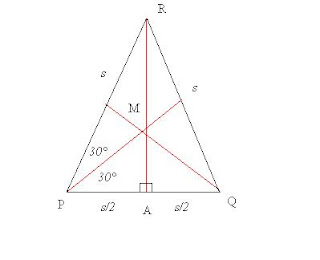## Wednesday, October 05, 2011

### Something simple

Well, maybe not so simple. But it was something I needed, and of course, since I could not lay my hand on a convenient reference, I decided to go through the exercise of finding out for myself.

What?

Nothing more exotic than the "central angle" of a tetrahedron.

So... first, let us take a regular tetrahedron...with its corners (vertices) labelled P,Q,R,S.

The length of all six edges is s. Each of its faces is an equilateral triangle with side s and angle 60°, or p/3 radians.

Here you see the face PQR with its altitudes shown in red:Note that the altitudes bisect the angles and the sides, and are perpendicular.

Since this is equilateral, and the altitude RA is the side opposite to the 60° angle with the hypotenuse of length s, the length of RA is given by:

|RA| = s*sqrt(3)/2

We also have a 30-60-90 triangle PMA, where the side opposite to 60° is s/2.

Each of the segments PM, QM, RM are the radii of the circumscribing circle of the triangle. Their lengths are the same, and is:

|PM| = s*sqrt(3)/3

The lengths of the segments from the center M to each edge is:

|AM| = s*sqrt(3)/6

As a check we see that

|RM| + |MA| = s*sqrt(3)/3 + s*sqrt(3)/6

or, since RM and MA are colinear,

|RA| = s*sqrt(3)/2

Now, let us go back to our tetrahedron and put in those medians in red, and some other lines from the center C to each vertex in green:We know that C is in a vertical line between M and S. Consider the triangle AMS, where A is the midpoint between P and Q. We know that it is a right triangle with its right angle at M; we also know (from above) the length of AS is s*sqrt(3)/2. We also know the length of AM, which is s*sqrt(3)/6, and so by the Pythagorean theorem, we can determine the altitude a of the tetrahedron, which is the length of SM:

|SM|2 + |AM|2 = |AS|2

Substituting, we get:

a2 + (s*sqrt(3)/6)2 = (s*sqrt(3)/2)2

This reduces to:

a = s*sqrt(6)/3

Next, we consider the triangle PCM. This has a right angle at M, and we know the length of PM to be s*sqrt(3)/3. We note that the length of PC is equal to that of SC - all these are the radii of the circumscribing sphere for the tetrahedron. We also note that the altitude SCM of the tetrahedron is colinear, so |SM| = |SC| + |CM|.

To simplfy things, let us call
r = |SC|

and
w = |CM|
, thus
r + w = a = s*sqrt(6)/3

Solving for w, we have:
w = a – r

Then, again by the Pythagorean theorem, we have:
r2 = w2 + |PM|2

Applying the value of |PM| and the above equation for w, we get:

r2 = (a – r)2 + (s*sqrt(3)/3)2

Now, it's just algebra to solve for r...

r2 = a22*r*a + r2 + (s2/3)

2*r*a = a2 + (s2/3)

but we also know that a = s*sqrt(6)/3, so we can reduce this to:

r = s*sqrt(6)/4

Recall that r is the radius of the circumscribing circle.

Now, consider the central triangle SCP, its two legs are radii and equal to r. The third leg is an edge of the tetrahedron which is given as s. Now, a line from C to the edge PS will bisect PS at a right angle. The central angle in SCP (at C) is twice the angle between that bisector and the radius PC, which means the desired angle is twice that which has a sine given by (s/2)/r. That is, the central angle q is given:

sin(q/2) = (s/2)/(s*sqrt(6)/4)

or
sin(q/2) = sqrt(6)/3)

As expected, the s term vanishes, since the central angle is independent of the size of the tetrahedron.

To complete the exercise, the angle q is approximately 109.471 degrees.

Wow. That was fun. Now, it's time to go and use that fact.

Maybe another day I'll tell you what it's for.

Oh yes. I expect that someone, somewhere is wondering whether this post has anything to do with yesterday's. As strange as it must sound, it does.

At 05 October, 2011 13:42,some guy on the street said...

Alternatively arguing from symmetry one might guess (though it's not a proof... yet) that the center --- the unique fixed point of the tetrahedral group acting on a solid tetrahedron --- is also the centroid --- informally, the mean of the four vertices. Without loss of generality we may take one vertex to lie on a coordinate axis at (r,0,0) (or, we may choose coordinates thus, and leave the tetrahedron where it is!) while the centroid is at (0,0,0). The other three vertices are at some point (r cos(θ),a,b) for varying values of a,b; but since the first coordinate of the centroid is zero, while it is also the mean of the four first coordintes, we find
3 cos(θ) + 1 = 0
or in other words, that
cos(θ) = -⅓.
Shall we do Morley's Miracle, next?

At 05 October, 2011 13:44,some guy on the street said...

(It's maybe not simple, but it *is* simplicial.)